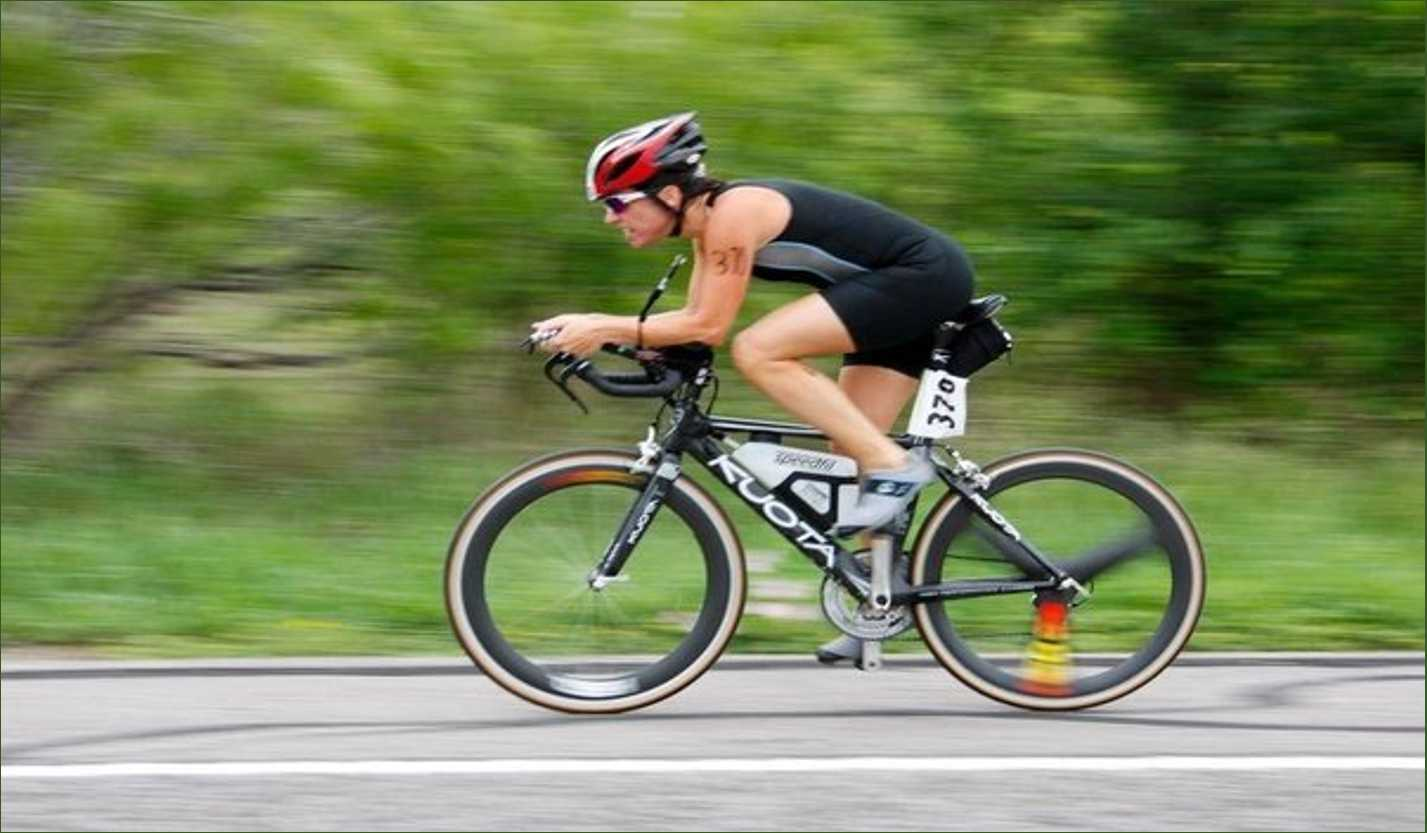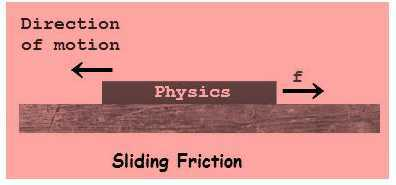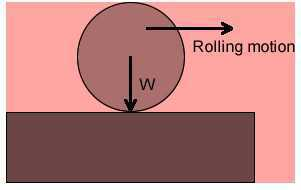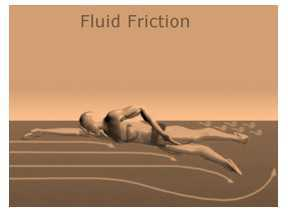Register for Maths, Science, English, Reasoning Olympiad Exams Register here | Book Free Demo for Live Olympiad Classes here| Check Olympiad Exam Dates here | Buy Practice Papers for IMO, IOM, HEO, IOEL etc here | Login here to participate in all India free mock test on every Saturday

# Friction## Force of friction

• The force which resists the relative motion of a surface is called as Friction.
• The force of friction is offered by all types of surface such as solid, liquid and the air.
• The force of friction is always applied in the opposite direction of the movement.
• When the two substance move towards each other, then friction is applied.

## Factors affecting friction

• When two surfaces are in contact then their irregularities cause friction.
• Increase in weight causes the increase in the force of friction.
• More force of friction is offered by Rougher surfaces.

## Types of Friction

1. Sliding friction
• When two surfaces overlaps then this force comes into action.
• Example is sliding of a box or book over the ground.1. Rolling friction
• When one surface rolls on the other surface then this friction comes to an action.
• Sliding friction is bigger than the rolling friction.## Why is friction necessary?

• Some examples which show friction is necessary are-
1. Due to the friction offered by ground, we can walk easily. Hence walking requires friction
2. Since wet marble floor or muddy tract offers very less amount of friction which makes difficult for us to walk easily on them.
3. Grooves on the soles of the shoes give good amount of friction to the ground which makes us walk easily due to the good grip.
4. Heat is also produced by friction.
5. Due to the friction provided by the paper to the tip of them pen, writing with the pen becomes possible.

### Fluid Friction

• Liquids and gases are generally termed as fluid. Fluids also provide friction just like the solids.
• With the speed of fluid and the speed of the moving object, the friction of fluid increases.
• When the fluids offer frictional force then that force is called as drag.## Recap

• The force which resists the relative motion of a surface is called as Friction.
• The force of friction is offered by all types of surface such as solid, liquid and the air.
• The force of friction is always applied in the opposite direction of the movement.
• When two surfaces are in contact then their irregularities cause friction.
• Liquids and gases are generally termed as fluid. Fluids also provide friction just like the solids.## Quiz for Friction

 Q.1 Define friction. a) A force that opposes relative motion b) A force that keeps an object moving c) A force which always increases weight d) balanced force of nature
 Q.2 Frictional forces act __________________ a) In similar direction of motion b) Nowhere c) Under two surfaces d) Between two surfaces in contact
 Q.3 Friction is dependent on _____________________. a) Climate b) Price of gold c) Nature of surface d) Election of the prime minister
 Q.4 Friction is a ____________________ force a) Artificial b) Natural c) Pessimistic d) Negative
 Q.5 A uniform chain of length L is lying on the horizontal surface of a table. If the coefficient of friction between the chain and the table top is μ. what is the maximum length of the chain that can hang over the edge of the table without disturbing the rest of the chain on table.? a) l=μL/(2+μ) b) l=μ/(1+μ) c) l=2μL/(1+μ) d) l=μL/(1+μ)
 Q.6 The coefficient of static and kinetic friction between a body and the surface are .75 and .50 respectively. A force is applied to the body to make it just slide with a constant acceleration which is a) a=g/8 b) a=g c) a=g/4 d) a=g/2
 Q.7 A block weighing 200 N is pushed along a surface. If it takes 80 N to get the block moving and 40 N to keep the block moving at a constant velocity, what are the coefficients of friction μs and μk? a) μs = 2.4, μk = 0.2. b) μs = 0.4, μk = 0.2. c) μs = 0.4, μk = 0.8. d) μs = 1.4, μk = 0.2.
 Q.8 A 5.0 kg Steel block is resting on a *horizontal table*. The coefficient of static friction (us) is 0.75 and the uk is 0.57. What minimum force is needed to start this block moving? a) 37 N b) 50 N c) 38 N d) 30 N
 Q.9 A 5.0 kg Steel block is resting on a *horizontal table*. The coefficient of static friction (us) is 0.75 and the uk is 0.57. What is the frictional force on this object as it moves? a) 18 N b) 28 N c) 38 N d) 48 N
 Q.10 A 5.0 kg Steel block is resting on a *horizontal table*. The coefficient of static friction (us) is 0.75 and the uk is 0.57. What force must be applied to the object to keep it moving at constant velocity? (Hint: Fnet = 0) a) 48 N b) 38 N c) 28 N d) 18 N